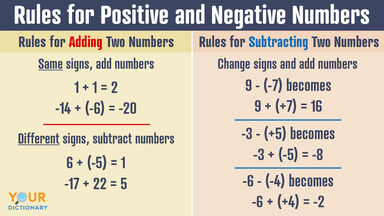# Basic Rules for Positive and Negative Numbers

Numbers higher than zero are called positive numbers, and numbers lower than zero are negative numbers. That means they fall at either side of the number line. However, just because they're on the same line doesn't mean they follow the same rules! Keep reading for a list of the basic rules for using positive and negative numbers in math.rules for adding and subtracting two numbers positive and negative

## Rules for Signed Numbers

When using positive and negative numbers, you use the rules for signed numbers (numbers with positive or negative signs in front of them). Also known as operations for signed numbers, these steps can help you avoid confusion and solve math problems as quickly — and correctly — as possible.

Follow these rules to determine the best way to add, subtract, multiply, and divide positive and negative numbers. Remember, if there is no + or - sign, the number is positive.

When you're adding two numbers together and they have the same sign (two positive or two negative numbers), add the numbers and keep the sign. For example:

• 1 + 1 = 2
• 51 + 32 = 83
• -14 + (-6) = -20
• -196 + (-71) = -267

Notice that equations with two positive numbers have positive sums, and equations with two negative numbers have negative sums. If you're using a number line to solve the problem, adding two positive numbers will go farther to the positive side, and adding two negative numbers will go farther on the negative side.

### Addition: Different Signs, Subtract the Numbers

If you're adding positive and negative numbers together, subtract the smaller number from the larger one and use the sign from the larger number. For example:

• 6 + (-5) = 1
• -17 + 22 = 5
• -100 + 54 = -45
• 299 + (-1) = 298

As you can see, adding numbers with different signs is really a form of subtraction. When using a number line, your sum will end up closer to zero.

Subtracting positive and negative numbers means that you add the opposite numbers, or additive inverse. Change the subtraction sign to addition and change the sign that follows to its opposite. Then follow the steps for addition. For example:

• -3 - (+5) becomes -3 + (-5) = -8
• 9 - (-7) becomes 9 + (+7) = 16
• -14 - (+8) becomes -14 + (-8) = -22
• 25 - (-90) becomes 25 + (+90) = 115

A good tip is that whenever you see a negative sign and a minus sign together, such as in 9 - (-7), immediately make them positive signs. The negative signs cancel each other out, and the equation becomes an addition problem.

### Multiplication and Division: Same Sign, Positive Result

It seems like multiplication and division would be more complicated than addition and subtraction, but they're actually much simpler. The rule for multiplying positive and negative numbers with the same sign (two positive or two negative) is that the product will always be positive. For example:

• 8 x 4 = 32
• (-8) x (-4) = 32
• 10 x 9 = 90
• (-10) x (-9) = 90

The same rule applies for division. When dividing a number by another number with the same sign, the quotient (answer) is positive. For example:

• 12 ÷ 6 = 2
• -12 ÷ (-6) = 2
• 100 ÷ 5 = 20
• -100 ÷ (-5) = 20

Why does multiplying or dividing two negative numbers always equal a positive number? Like subtracting negative numbers, these operations turn the negatives into their opposite (inverse). You are essentially subtracting the negative number several times — and as seen above, subtracting negative numbers results in a positive equation.

### Multiplication and Division: Opposite Sign, Negative Result

When multiplying a positive and a negative, the product will always be negative. It doesn't matter what order the signs are in. For example:

• 6 x (-7) = -42
• -7 x 6 = -42
• 12 x (-11) = -132
• -11 x 12 = -132

In all of these cases, you first need to multiply or divide the numbers. Then decide whether the product or quotient is positive (two positives or two negatives in the equation) or negative (one positive and one negative in the equation).

## Like and Unlike Signs in Addition and Subtraction

Another way to think about adding positive and negative numbers is to look at the signs in a row. Two like signs in a row (++ or --) mean you add the numbers, while two unlike signs in a row (+- or -+) mean that you subtract. For example:

• 7 + (+2) = 9 (++ are like signs, so the equation is addition)
• 9 + (-8) = 1 (+- are unlike signs, so the equation is subtraction)
• 11 - (+13) = 2 (-+ are unlike signs, so the equation is subtraction)
• 15 - (-10) = 25 (-- are like signs, so the equation is addition)

This method follows the same rules as above but might help you solve the problem more quickly if you prefer to work out the signs beforehand. Once you understand positive and negative numbers conceptually, you can decide which method works best for you.

## Understanding Math Foundations

Once you know the basics of math and its rules, the entire mathematical world is open to you. Unlike other subjects, math isn't nuanced or up for interpretation — it just is what it is! For more math practice, check out the steps to long division problems (with examples). You can also review the different types of numbers in math before your next math assignment.